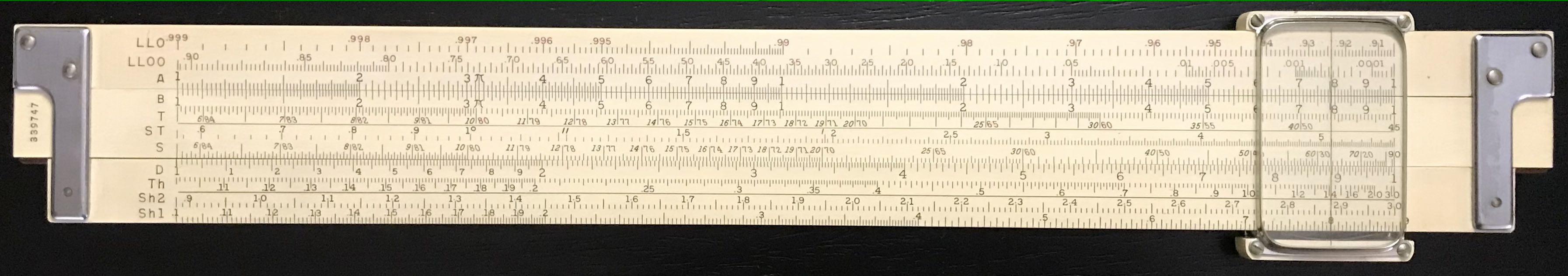## 3.9 Hyperbolic Trigonometric Scales

On a select number of slide rules, scales of values of the hyperbolic trigonometric functions might be found. These scales, typically labeled SH and TH (or Sh and Th), operate much like the normal trigonometric scales S and T. The physical length from the index to a value $$x$$ on the SH scale, for instance, is proportional to the logarithm of the value of the function $$\sinh(x)$$. The hyperbolic trig functions are defined in terms of the exponential function:

$\sinh x = \frac{e^x - e^{-x}}{2} ~~~~~~~~~~~~ \cosh x = \frac{e^x + e^{-x}}{2} \\ \tanh x = \frac{e^x - e^{-x}}{e^x + e^{-x}}$

Again, like the basic trig functions, the $$\cosh$$ function is typically not found on the slide rule; it can be computed from $$\cosh x$$ = $$\sinh x/\tanh x$$.K&E Model 4083-3 Vector Rule (back).

The hyperbolic trig functions appear in computations such as in the case of a rope attached at two ends and hanging under its own gravity (called a catenary), or in various computations that entail exponential growth or decay. For instance, from the above definitions it can be seen that

$e^x = \cosh x + \sinh x, ~~~~~~~~ {\rm and} ~~~~~~~~ e^{-x} = \cosh x - \sinh x.$

In addition, sometimes problems require the calculation of trigonometric functions of complex numbers. A complex number has a real and an imaginary component. The result of such an operation results in a new complex number with its own real and imaginary components, and these components will depend upon hyperbolic functions of the original components. It is actually this particular application for which slide rules with the hyperbolic functions were first created, primarily for use in electrical engineering. As this is a very special use of the hyperbolic functions, further discussion of these so-called “vector” calculations is reserved for the vignette Vector/Hyperbolic Calculations.